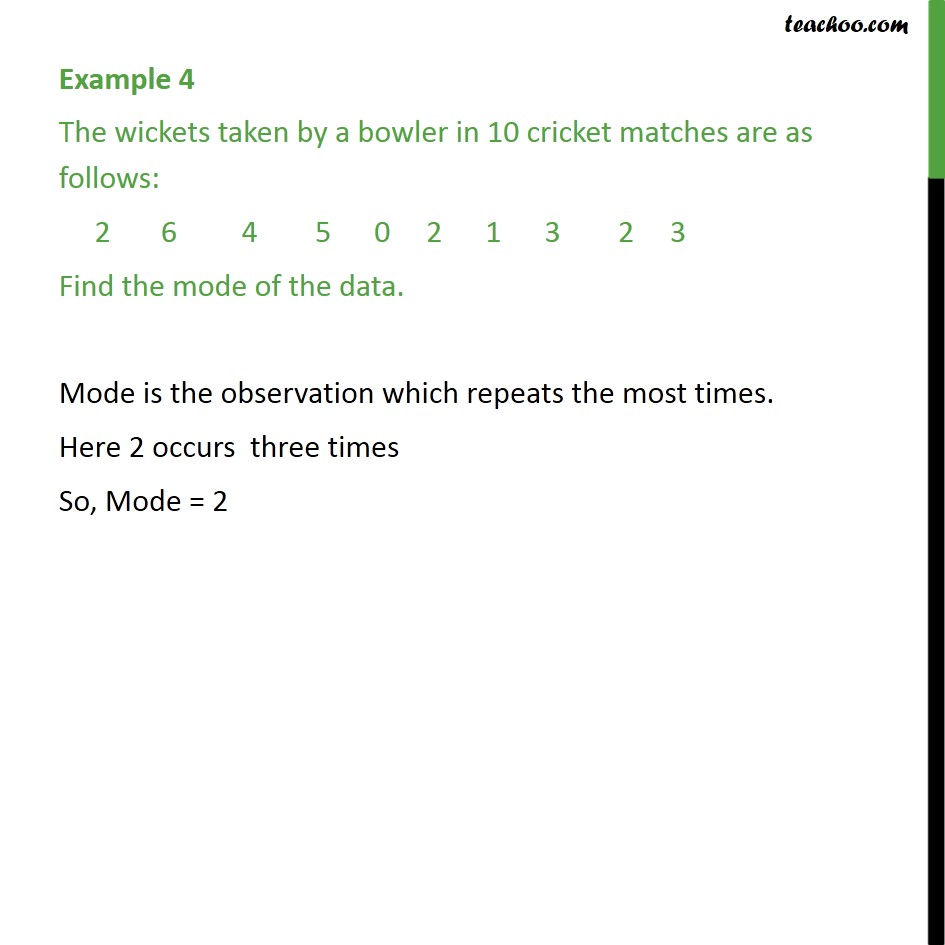1. Chapter 14 Class 10 Statistics
2. Serial order wise
3. Examples

Transcript

Example 4 The wickets taken by a bowler in 10 cricket matches are as follows: 2 6 4 5 0 2 1 3 2 3 Find the mode of the data. In this question, we need to find Mode. We know that Mode is the observation which repeats the most times. So, we find the observation which occurs most number of times Here, 2 occurs three times So, Mode = 2

Examples

Chapter 14 Class 10 Statistics
Serial order wise